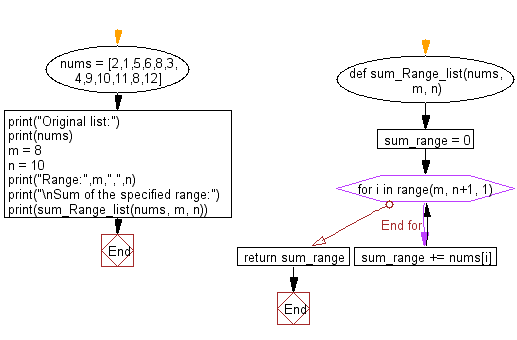﻿ Python: Sum of the numbers in a list between the indices of a specified range - w3resource# Python: Sum of the numbers in a list between the indices of a specified range

## Python List: Exercise - 128 with Solution

Write a Python program to calculate the sum of the numbers in a list between the indices of a specified range.

Sample Solution:

Python Code:

``````def sum_Range_list(nums, m, n):
sum_range = 0
for i in range(m, n+1, 1):
sum_range += nums[i]
return sum_range

nums = [2,1,5,6,8,3,4,9,10,11,8,12]
print("Original list:")
print(nums)
m = 8
n = 10
print("Range:",m,",",n)
print("\nSum of the specified range:")
print(sum_Range_list(nums, m, n))
```
```

Sample Output:

```Original list:
[2, 1, 5, 6, 8, 3, 4, 9, 10, 11, 8, 12]
Range: 8 , 10

Sum of the specified range:
29
```

Flowchart:## Visualize Python code execution:

The following tool visualize what the computer is doing step-by-step as it executes the said program:

Python Code Editor:

Have another way to solve this solution? Contribute your code (and comments) through Disqus.

What is the difficulty level of this exercise?

Test your Python skills with w3resource's quiz

﻿

## Python: Tips of the Day

Floor Division:

When we speak of division we normally mean (/) float division operator, this will give a precise result in float format with decimals.

For a rounded integer result there is (//) floor division operator in Python. Floor division will only give integer results that are round numbers.

```print(1000 // 300)
print(1000 / 300)```

Output:

```3
3.3333333333333335```